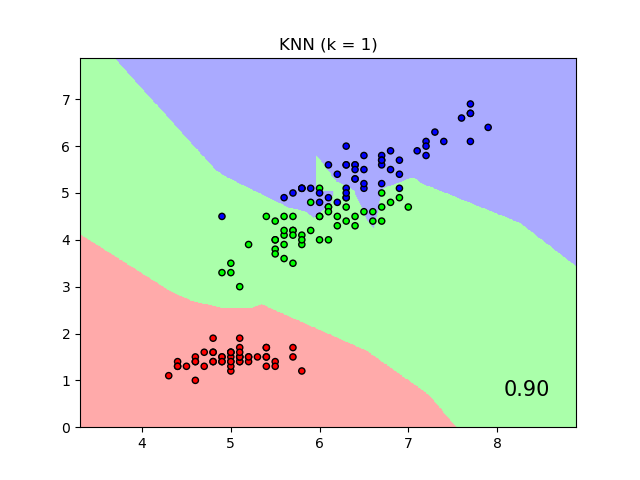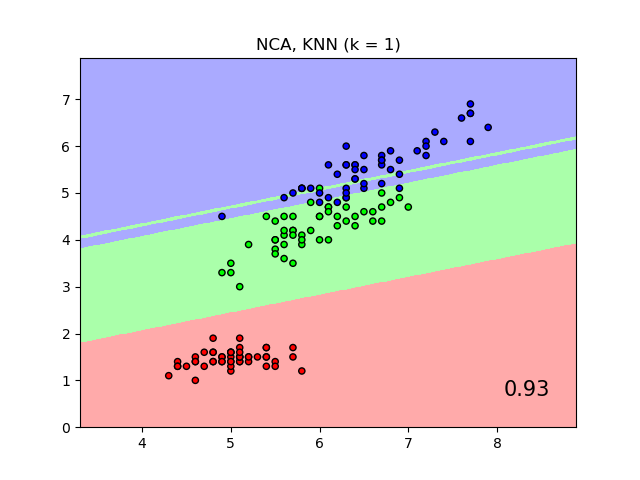# Comparing Nearest Neighbors with and without Neighborhood Components Analysis¶

An example comparing nearest neighbors classification with and without Neighborhood Components Analysis.

It will plot the class decision boundaries given by a Nearest Neighbors classifier when using the Euclidean distance on the original features, versus using the Euclidean distance after the transformation learned by Neighborhood Components Analysis. The latter aims to find a linear transformation that maximises the (stochastic) nearest neighbor classification accuracy on the training set.

••# License: BSD 3 clause

import numpy as np
import matplotlib.pyplot as plt
from matplotlib.colors import ListedColormap
from sklearn import datasets
from sklearn.model_selection import train_test_split
from sklearn.preprocessing import StandardScaler
from sklearn.neighbors import (KNeighborsClassifier,
NeighborhoodComponentsAnalysis)
from sklearn.pipeline import Pipeline

print(__doc__)

n_neighbors = 1

X, y = dataset.data, dataset.target

# we only take two features. We could avoid this ugly
# slicing by using a two-dim dataset
X = X[:, [0, 2]]

X_train, X_test, y_train, y_test = \
train_test_split(X, y, stratify=y, test_size=0.7, random_state=42)

h = .01  # step size in the mesh

# Create color maps
cmap_light = ListedColormap(['#FFAAAA', '#AAFFAA', '#AAAAFF'])
cmap_bold = ListedColormap(['#FF0000', '#00FF00', '#0000FF'])

names = ['KNN', 'NCA, KNN']

classifiers = [Pipeline([('scaler', StandardScaler()),
('knn', KNeighborsClassifier(n_neighbors=n_neighbors))
]),
Pipeline([('scaler', StandardScaler()),
('nca', NeighborhoodComponentsAnalysis()),
('knn', KNeighborsClassifier(n_neighbors=n_neighbors))
])
]

x_min, x_max = X[:, 0].min() - 1, X[:, 0].max() + 1
y_min, y_max = X[:, 1].min() - 1, X[:, 1].max() + 1
xx, yy = np.meshgrid(np.arange(x_min, x_max, h),
np.arange(y_min, y_max, h))

for name, clf in zip(names, classifiers):

clf.fit(X_train, y_train)
score = clf.score(X_test, y_test)

# Plot the decision boundary. For that, we will assign a color to each
# point in the mesh [x_min, x_max]x[y_min, y_max].
Z = clf.predict(np.c_[xx.ravel(), yy.ravel()])

# Put the result into a color plot
Z = Z.reshape(xx.shape)
plt.figure()
plt.pcolormesh(xx, yy, Z, cmap=cmap_light, alpha=.8)

# Plot also the training and testing points
plt.scatter(X[:, 0], X[:, 1], c=y, cmap=cmap_bold, edgecolor='k', s=20)
plt.xlim(xx.min(), xx.max())
plt.ylim(yy.min(), yy.max())
plt.title("{} (k = {})".format(name, n_neighbors))
plt.text(0.9, 0.1, '{:.2f}'.format(score), size=15,
ha='center', va='center', transform=plt.gca().transAxes)

plt.show()


Total running time of the script: ( 0 minutes 16.006 seconds)

Gallery generated by Sphinx-Gallery